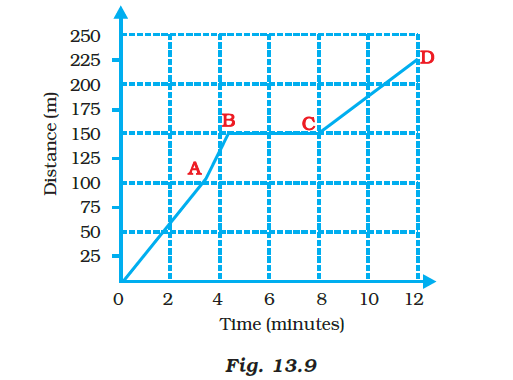# Boojho goes to the football ground to play football. The distance-time graph of his journey from his home to the ground is given in the Figure (a) What does the graph between point B and C indicate about the motion of Boojho? (b) Is the motion between 0 to 4 minutes uniform or nonuniform? (c) What is his speed between 8 and 12 minutes of his journey?(a) As time changes, the graph between points B and C is parallel to the x-axis, indicating that there is no motion. Boojho is in a rest state.

(b) Because the graph is not straight, the motion from 0 to 4 minutes is nonuniform.

(c)

At time 12-minute distance = 225 m

At time 8-minute distance = 150 m

Speed is distance divided by the time taken.

S = D/t

S = (225 – 150)/(12 – 8)

S = 75/4

S = 18.75 m/min(0)(0)